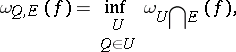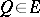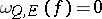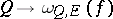# Oscillation of a function

(diff) ← Older revision | Latest revision (diff) | Newer revision → (diff)on a setThe difference between the least upper and the greatest lower bounds of the values ofon. In other words, the oscillation ofonis given byIf the function is unbounded on, its oscillation onis put equal to. For constant functions on(and only for these) the oscillation onis zero. If the functionis defined on a subsetof, then its oscillation at any pointof the closure ofis defined by the formulawhere the infimum is taken over all neighbourhoodsof. If, then in order thatbe continuous atwith respect to the setit is necessary and sufficient that.

The functionis called the oscillation function of.